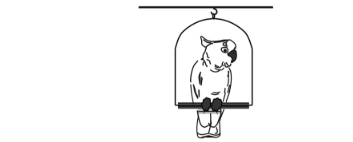# Problem: The bird perched on the swing in the figure has a mass of 44 g, and the base of the swing has a mass of 136.4 g. Assume that the swing and the bird are originally at rest and that the bird then takes off horizontally at 2.74 m/s. The acceleration of gravity is 9.8 m/s2. If the base can swing freely (without friction) around the pivot, how high will the base of the swing rise above its original level? 1. 0.0267539 2. 0.0357149 3. 0.0398586 4. 0.025 5. 0.0312608 6. 0.0442463 7. 0.0282227 8. 0.0329453 9. 0.0410105 10. 0.0352356

###### FREE Expert Solution
83% (191 ratings)
###### Problem Details

The bird perched on the swing in the figure has a mass of 44 g, and the base of the swing has a mass of 136.4 g. Assume that the swing and the bird are originally at rest and that the bird then takes off horizontally at 2.74 m/s. The acceleration of gravity is 9.8 m/s2. If the base can swing freely (without friction) around the pivot, how high will the base of the swing rise above its original level?

1. 0.0267539

2. 0.0357149

3. 0.0398586

4. 0.025

5. 0.0312608

6. 0.0442463

7. 0.0282227

8. 0.0329453

9. 0.0410105

10. 0.0352356